# Finding the base

Find the unknown base of percent:

12.5 percent of what = 16?

Correct result:

x =  128

#### Solution:

12.5/100 • x = 16

12.5x = 1600

x = 128

Our simple equation calculator calculates it.We would be pleased if you find an error in the word problem, spelling mistakes, or inaccuracies and send it to us. Thank you!Tips to related online calculators
Do you have a linear equation or system of equations and looking for its solution? Or do you have quadratic equation?

## Next similar math problems:

• Unknown number 716% of the unknown number is by 21 less than unknown number itself. Determine the natural unknown number.
• Unknown number x5% from an unknown number equals the number 26. What is the unknown number?
• Original planGardeners planted 16% more currant bushes than originally planned. In total, they planted x = 435 shrubs. What was the original plan?
• The base 2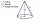The base diameter of a right cone is 16cm and it's slant height is 12cm. A. ) Find the perpendicular height of the cone to 1 decimal place. B. ) Find the volume of the cone, convert to 3 significant figure. Take pie =3.14
• After 16 years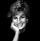After 16 years will Dana be five times older as she was four years ago. After how many years will Dana celebrate her 16th birthday?
• Unknown number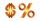15% of the unknown number is 18 less than 21% of the same number. What is the unknown number?
• Vinegar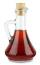What percentage vinegar we get if we mix 1 if dm³ eight percent vinegar with 1.5 dm³ six percent vinegar?
• Isosceles trapezoidFind the area of an isosceles trapezoid, if the bases are 12 cm and 20 cm, the length of the arm is 16 cm
• 14 percentIf 14 percent is 56, what is the base?
• Unknown mixed numberFind the number that is smaller than 5 5/12 by as much as 2 2/13 is smaller than 6 1/6
• Percentage reduction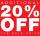Reducing the unknown number by 19% get number 700.7 Determine unknown number.
• The circumferenceThe circumference and width of the rectangle are in a ratio of 5: 1. its area is 216cm2. What is its length?
• KLMN trapezoidThe KLMN trapezoid has bases KL 40cm and MN 16cm. On the KL base is point P. The segment NP divides the trapezoid into units with the same area. What is the distance of point P from point K?
• Reducing numberReducing the an unknown number by 28.5% we get number 243.1. Determine unknown number.
• Unknown number 24f we add 20, we get 50% of its triple. What is this unknown number?
• The ropeA 68 centimetre long rope is used to make a rhombus on the ground. The distance between a pair of opposite side corners is 16 centimetres what is the distance between the other two corners?
• Bamboo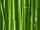Bamboo high 32 feet was at a certain height broken by the wind so the bamboo top reached the ground at a distance of 16 feet from the trunk. At what height from the ground was the bamboo broken?